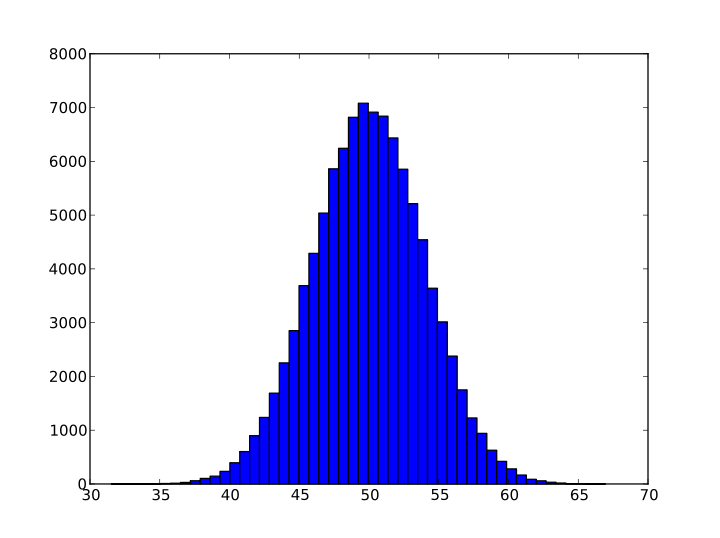# This note has been used to help create the Normal Distribution wikiBegin with a row from the Pascal triangle, preferably some large exponent , derive the Gaussian Distribution.

Solution

The bell curve is a probability density curve of binary systems. Then the probability at a some displacement from the medium is $P(n, k) = \left( \begin{matrix} n \\ k \end{matrix} \right) {2}^{-n}= \frac{n!}{(\frac{1}{2}n + k)! (\frac{1}{2}n - k)! {2}^{n}}$

Using the Stirling approximation and treating $k = \frac{\sigma}{2}$, we have
$P(n, \sigma) \sim {\left(\frac{n}{2\pi} \right)}^{\frac{1}{2}} {\left(\frac{n}{2}\right)}^{n} {\left(\frac{{n}^{2} - {\sigma}^{2}}{4}\right)}^{-\frac{1}{2}(n+1)}{\left(\frac{n + \sigma}{n-\sigma}\right)}^{\frac{-\sigma}{2}}.$

For $n>>\sigma$, $\frac{n + \sigma}{n-\sigma} \sim 1+\frac{2\sigma}{n}$; hence, for large $n$ $P(n, \sigma) \sim {\left(\frac{n}{2\pi} \right)}^{\frac{1}{2}} {\left(1- \frac{{\sigma}^{2}}{{n}^{2}}\right)}^{-\frac{1}{2}(n+1)}{\left(1+\frac{2\sigma}{n}\right)}^{\frac{-\sigma}{2}}.$

Taking the logarithm yields $ln(P(n,\sigma)) \sim \frac{1}{2}ln \left (\frac{2}{\pi n}\right) - \frac{1}{2}(n+1)ln \left (1- \frac{{\sigma}^{2}}{{n}^{2}}\right) - \frac{\sigma}{2}ln \left (1+\frac{2\sigma}{n}\right).$

For small $x$, $ln(1+x) \approx x$; subsequently, $ln(P(n,\sigma)) \sim \frac{1}{2}ln \left (\frac{2}{\pi n}\right) - \frac{1}{2}(n+1) \left (-\frac{{\sigma}^{2}}{{n}^{2}}\right) - \frac{\sigma}{2} \left (\frac{2\sigma}{n}\right)$ or $ln(P(n,\sigma)) \sim \frac{1}{2}ln \left (\frac{2}{\pi n}\right) + \frac{{\sigma}^{2}}{{n}^{2}} - \frac{{\sigma}^{2}}{2n}.$

Since $\frac{{\sigma}^{2}}{{n}^{2}}$ vanishes faster than $\frac{{\sigma}^{2}}{2n}$ for very large $n$, we arrive at the result:

$P(n, \sigma) = {\left(\frac{2}{\pi n} \right)}^{\frac{1}{2}} {e}^{\frac{-{\sigma}^{2}}{2n}}.$

Check out my other notes at Proof, Disproof, and DerivationNote by Steven Zheng
6 years, 10 months ago

This discussion board is a place to discuss our Daily Challenges and the math and science related to those challenges. Explanations are more than just a solution — they should explain the steps and thinking strategies that you used to obtain the solution. Comments should further the discussion of math and science.

When posting on Brilliant:

• Use the emojis to react to an explanation, whether you're congratulating a job well done , or just really confused .
• Ask specific questions about the challenge or the steps in somebody's explanation. Well-posed questions can add a lot to the discussion, but posting "I don't understand!" doesn't help anyone.
• Try to contribute something new to the discussion, whether it is an extension, generalization or other idea related to the challenge.
• Stay on topic — we're all here to learn more about math and science, not to hear about your favorite get-rich-quick scheme or current world events.

MarkdownAppears as
*italics* or _italics_ italics
**bold** or __bold__ bold
- bulleted- list
• bulleted
• list
1. numbered2. list
1. numbered
2. list
Note: you must add a full line of space before and after lists for them to show up correctly
paragraph 1paragraph 2

paragraph 1

paragraph 2

[example link](https://brilliant.org)example link
> This is a quote
This is a quote
    # I indented these lines
# 4 spaces, and now they show
# up as a code block.

print "hello world"
# I indented these lines
# 4 spaces, and now they show
# up as a code block.

print "hello world"
MathAppears as
Remember to wrap math in $$ ... $$ or $ ... $ to ensure proper formatting.
2 \times 3 $2 \times 3$
2^{34} $2^{34}$
a_{i-1} $a_{i-1}$
\frac{2}{3} $\frac{2}{3}$
\sqrt{2} $\sqrt{2}$
\sum_{i=1}^3 $\sum_{i=1}^3$
\sin \theta $\sin \theta$
\boxed{123} $\boxed{123}$

## Comments

There are no comments in this discussion.

×

Problem Loading...

Note Loading...

Set Loading...